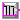## Buildings.Controls.OBC.CDL.Logical.Sources

Package with blocks that generate source signals

### Information

Package with blocks that generate signals.

### Package Content

Name DescriptionConstant Output constant signal of type BooleanPulse Generate pulse signal of type BooleanSampleTrigger Generate sample trigger signalTimeTable Table look-up with respect to time with constant segmentsValidation Collection of models that validate the logical sources blocks of the CDL

##Buildings.Controls.OBC.CDL.Logical.Sources.Constant

Output constant signal of type Boolean### Information

Block that outputs a constant signal `y = k`, where `k` is a Boolean-valued parameter.### Parameters

TypeNameDefaultDescription
Booleank Constant output value

### Connectors

TypeNameDescription
output BooleanOutputyConnector of Boolean output signal

### Modelica definition

block Constant "Output constant signal of type Boolean" parameter Boolean k "Constant output value"; Interfaces.BooleanOutput y "Connector of Boolean output signal"; equation y=k; end Constant;

##Buildings.Controls.OBC.CDL.Logical.Sources.Pulse

Generate pulse signal of type Boolean### Information

Block that outputs a pulse signal as shown below.The pulse signal is generated an infinite number of times, and aligned with `time=shift`.

### Parameters

TypeNameDefaultDescription
Realwidth0.5Width of pulse in fraction of period 
Realperiod Time for one period [s]
Realshift0Shift time for output [s]

### Connectors

TypeNameDescription
output BooleanOutputyConnector of Boolean output signal

### Modelica definition

block Pulse "Generate pulse signal of type Boolean" parameter Real width( final min=Constants.small, final max=1, final unit="1")=0.5 "Width of pulse in fraction of period"; parameter Real period( final quantity="Time", final unit="s", final min=Constants.small) "Time for one period"; parameter Real shift( final quantity="Time", final unit="s")=0 "Shift time for output"; Interfaces.BooleanOutput y "Connector of Boolean output signal"; protected parameter Real t0( final quantity="Time", final unit="s", fixed=false) "First sample time instant"; parameter Real t1( final quantity="Time", final unit="s", fixed=false) "First end of amplitude"; initial algorithm t0 := Buildings.Utilities.Math.Functions.round( x=integer((time)/period)*period+mod(shift,period), n=6); t1 := t0+width*period; // Make sure t0 and t1 are within time + period. if time+period < t1 then t0 := t0-period; t1 := t1-period; end if; // Make sure time is between t0 and t1, or t1 and t0 // Now, t0 < t1 if time >= t1 then t0 := t0+period; elseif time < t0 then t1 := t1-period; end if; // Assert that t0 <= t < t1 or t1 <= t < t0 if(t0 < t1) then assert( t0 <= time and time < t1, getInstanceName()+": Implementation error in initial time calculation: t0 = "+String(t0)+", t1 = "+String(t1)+", period = "+String(period)+", time = "+String(time)); y := time >= t0 and time < t1; else assert( t1 <= time and time < t0, getInstanceName()+": Implementation error in initial time calculation: t0 = "+String(t0)+", t1 = "+String(t1)+", period = "+String(period)+", time = "+String(time)); y := not(time >= t1 and time < t0); end if; equation when sample( t0, period) then y=true; elsewhen sample( t1, period) then y=false; end when; end Pulse;

##Buildings.Controls.OBC.CDL.Logical.Sources.SampleTrigger

Generate sample trigger signal### Information

The Boolean output `y` is a trigger signal that is only `true` at sample times (defined by parameter `period`) and is otherwise `false`.The trigger signal is generated an infinite number of times, and aligned with `time=delay`.

### Parameters

TypeNameDefaultDescription
Realperiod Sample period [s]
Realshift0Shift time for output [s]

### Connectors

TypeNameDescription
output BooleanOutputyConnector of Boolean output signal

### Modelica definition

block SampleTrigger "Generate sample trigger signal" parameter Real period( final quantity="Time", final unit="s", final min=Constants.small) "Sample period"; parameter Real shift( final quantity="Time", final unit="s")=0 "Shift time for output"; Interfaces.BooleanOutput y "Connector of Boolean output signal"; protected parameter Real t0( final quantity="Time", final unit="s", fixed=false) "First sample time instant"; initial equation t0=Buildings.Utilities.Math.Functions.round( x=integer((time)/period)*period+mod(shift,period), n=6); equation y=sample( t0, period); end SampleTrigger;

##Buildings.Controls.OBC.CDL.Logical.Sources.TimeTable

Table look-up with respect to time with constant segments### Information

Block that outputs `true`/`false` time table values.

The block takes as a parameter a time table of a format:

```table = [ 0*3600, 0;
6*3600, 1;
6*3600, 0;
18*3600, 1;
18*3600, 1];
period = 24*3600;
```

where the first column of `table` is time and the remaining column(s) are the table values. The time column contains `Real` values that are in units of seconds if `timeScale = 1`. The parameter `timeScale` can be used to scale the time values, for example, use `timeScale = 3600` if the values in the first column are interpreted as hours.

The values in column two and higher must be `0` or `1`, otherwise the model stops with an error.

Until a new tabulated value is set, the previous tabulated value is returned.

The table scope is repeated periodically with periodicity `period`.

### Parameters

TypeNameDefaultDescription
Realtable[:, :] Table matrix with time as a first column (in seconds, unless timeScale is not 1) and 0 for False or 1 for True in all other columns
RealtimeScale1Time scale of first table column. Set to 3600 if time in table is in hours 
Realperiod Periodicity of table [s]

### Connectors

TypeNameDescription
output BooleanOutputy[nout]Output of the table

### Modelica definition

block TimeTable "Table look-up with respect to time with constant segments" parameter Real table[:,:] "Table matrix with time as a first column (in seconds, unless timeScale is not 1) and 0 for False or 1 for True in all other columns"; parameter Real timeScale( final unit="1")=1 "Time scale of first table column. Set to 3600 if time in table is in hours"; parameter Real period( final quantity="Time", final unit="s") "Periodicity of table"; Interfaces.BooleanOutput y[nout] "Output of the table"; protected final parameter Integer nout=size( table, 2)-1 "Dimension of output vector"; final parameter Integer nT=size( table, 1) "Number of table points"; Integers.Sources.TimeTable intTimTab( final table=table, final timeScale=timeScale, final period=period) "Time table"; Integers.GreaterThreshold intGreThr[nout]( each t=0) "Converts to boolean"; initial equation // Check that all values in the second column are Integer values for i in 1:nT loop for j in 2:size( table, 2) loop assert( (abs( table[i,j]) < Constants.small) or (abs( table[i,j]-1.0) < Constants.small), "Table value table["+String(i)+", "+String(j)+"] = "+String( table[i,j])+" does not equal either 0 or 1."); end for; end for; equation connect(intTimTab.y,intGreThr.u); connect(intGreThr.y,y); end TimeTable;